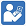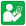Test Sheets - Multiply 2-Digit Numbers
Multiply 2-Digit Numbers - Test 1
Multiply 2-Digit Numbers - Test 2
Multiply 2-Digit Numbers - Test 3Practice Sheets - Multiply 2-Digit Numbers